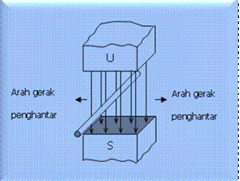Home > English > Directions Elektromotive Style

# Directions Elektromotive Style

The direction of the electromotive force (EMF / electromotive force) generated on a conductor in a magnetic field will vary according to the change in direction of the magnetic flux and the direction to which the conductor moves. If the conductor is moved (in the direction shown by the big arrow in Figure 4 between the north and south magnetic poles, the electromotive force will flow from right to left (the direction of the magnetic flux is from the north pole to the south pole.) The direction of the electromotive force can be understood by using Fleming’s Right Hand Rule.

With the thumb, forefinger and middle finger of one’s right hand stretched by the angle of the elbow between one another (Fig. 1.11), then the index finger will indicate the direction of the magnetic flux (the magnetic force line), the thumb is the direction of the conductor movement, and the middle finger is the direction of the electromotive force.

B. Number of Electromotive StylesFigure 1.12 Electromotive force

The amount of eletromotive force generated when the conductor intersects (through) the magnetic flux inside a magnetic field is proportional to the number of lines of magnetic force cut off within a given time unit Fig. 1.12. For example, if a number of lines are cut in seconds (t) and the electromotive force is volt (E), then this can be expressed in the following formula:
E = Blv
Where :
l = Length of wire / meter in meters.
B = Large magnetic induction in W / m2 or Tesla. v = Speed ​​of carriage in m / s.
E = Induced electric force (induction) in volts."Tasikmalaya, Indonesia"